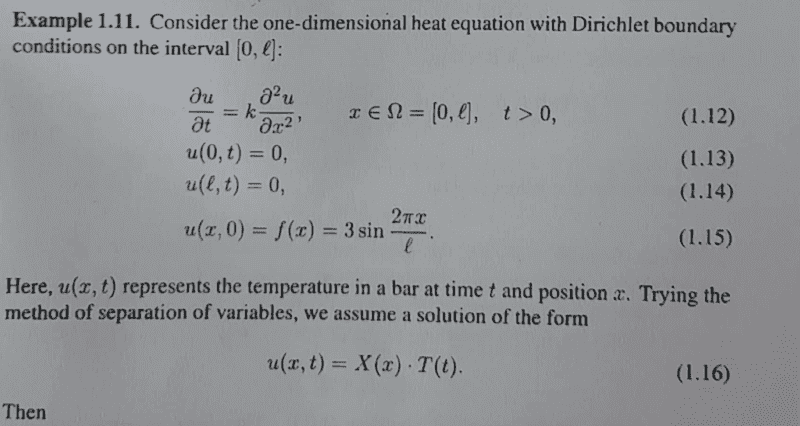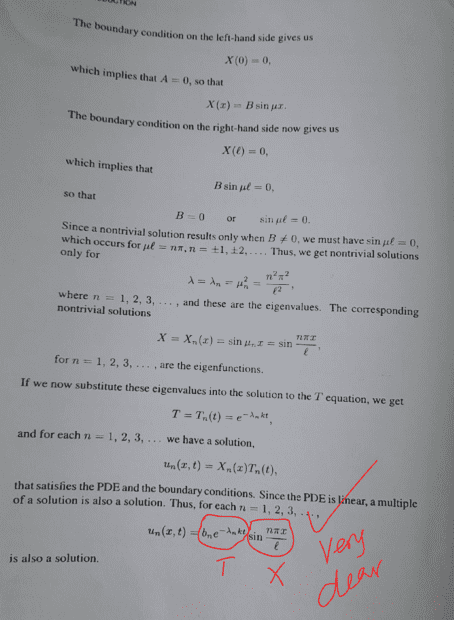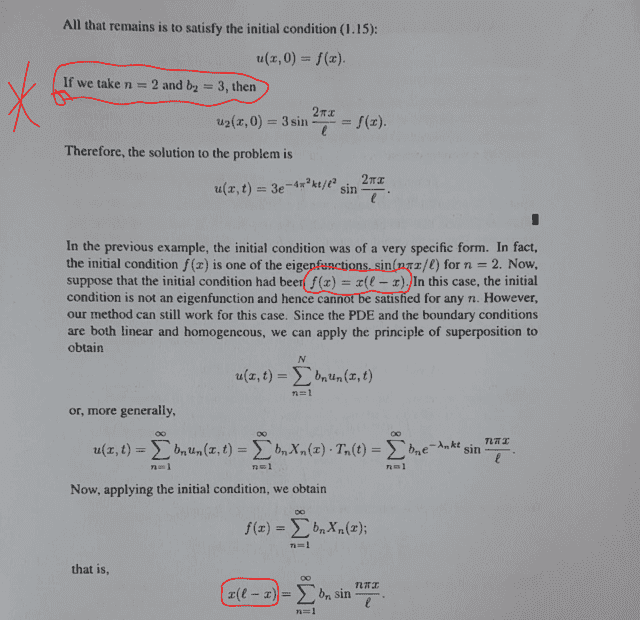# Solve the heat equation having Dirichlet boundary conditions

• A
• chwala
In summary, if you are solving for a non-eigen function initial condition, you will need to use the principle of superposition and use the appropriate boundary conditions.

#### chwala

Gold Member
TL;DR Summary
See attached.
I am going through these notes...they are pretty easy to follow. I would like more insight on the initial condition. In this problem, (attachment below), i guess the choice of initial condition is convenient as its easier to plug in the values of ##n=2## and ##b=3## (highlighted on the attachment) to realize the required solution. My question is? supposing we had the initial condition being;

##f(x)=\dfrac{1}{2}\sin\left[\dfrac{4πx}{l}\right]## where then our ##n=4## and ##b_4=\dfrac{1}{2}##

then our solution would be;

##U(x,t)##= ##\dfrac{1}{2} e^{\left[\dfrac{-16π^2kt}{l^2}\right]} ×\sin\left[ \dfrac{4πx}{l}\right]## correct?

I think the other highlighted part is now clear to me i.e when the initial condition is not an eigen function, then we have to make use of the principle of superposition. (one thing that is clear to me here is that for us to have the eigen function then the initial condition has to be either a function of sine or cosine).

Can we have the initial condition being a non-trigonometric function which would give us an eigenfunction?

Cheers!

Find the problem and solution here from textbook;Last edited:
The operator $X'' + \lambda X$ is self-adjoint with respect to the inner product $$\langle f, g \rangle = \int_0^\ell f(x)g(x)\,dx,$$ so eigenfunctions corresponding to different eigenvalues will be orthogonal with respect to this inner product, and you can recover the $b_n$ from $$\int_0^{\ell} f(x)X_m(x)\,dx = b_m \int_0^{\ell} X_m(x)^2\,dx$$ as long as you can do the integrals.

•chwala
pasmith said:
The operator $X'' + \lambda X$ is self-adjoint with respect to the inner product $$\langle f, g \rangle = \int_0^\ell f(x)g(x)\,dx,$$ so eigenfunctions corresponding to different eigenvalues will be orthogonal with respect to this inner product, and you can recover the $b_n$ from $$\int_0^{\ell} f(x)X_m(x)\,dx = b_m \int_0^{\ell} X_m(x)^2\,dx$$ as long as you can do the integrals.
Thanks @pasmith . Let me read more on this.

chwala said:
Summary: See attached.

I am going through these notes...they are pretty easy to follow. I would like more insight on the initial condition. In this problem, (attachment below), i guess the choice of initial condition is convenient as its easier to plug in the values of ##n=2## and ##b=3## (highlighted on the attachment) to realize the required solution. My question is? supposing we had the initial condition being;

##f(x)=\dfrac{1}{2}\sin\left[\dfrac{4πx}{l}\right]## where then our ##n=4## and ##b_4=\dfrac{1}{2}##

then our solution would be;

##U(x,t)##= ##\dfrac{1}{2} e^{\left[\dfrac{-16π^2kt}{l^2}\right]} ×\sin\left[ \dfrac{4πx}{l}\right]## correct?

I think the other highlighted part is now clear to me i.e when the initial condition is not an eigen function, then we have to make use of the principle of superposition. (one thing that is clear to me here is that for us to have the eigen function then the initial condition has to be either a function of sine or cosine).

Can we have the initial condition being a non-trigonometric function which would give us an eigenfunction?

Cheers!

Find the problem and solution here from textbook;
View attachment 314544

View attachment 314545
View attachment 314546
I have been doing further reading on this by looking at heat diffusivity on insulated wire. I have noted that in general if the initial condition ##f(x)## is a sine series then the homogenous Dirichlet boundary conditions will apply for insulated wire (with non-insulated ends) resulting into a Fourier series of the odd periodic extension ##f(x)##. I have also noted that for an insulated wire (with insulated ends), the homogenous Neumann boundary conditions will apply and the ensuing solution would be Fourier series of the even peridic extension ##f(x)##...